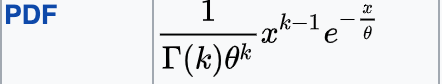# Gamma distribution negative log-likelihood minimization error

Hi,

I am trying to fit a gamma distribution over real-values data, in a regression setting by using LSTM over the time-series input.

I am predicting the k and theta parameterfrom the last state of LSTM over input sequence.

I am using the following NLL function:

def gamma_nll(inp, target):

``````target = torch.squeeze(target, 1)

eps = 1e-8
# inp is a 2 dim param vector for weibull distribution

k = neta[:, 0]
theta = neta[:, 1]

log_gamma = -2.081061466 - k + 0.0833333 / (3.0 + k + eps) - torch.log(k * (1.0 + k) * (2.0 + k) + eps) + (2.5 + k) * torch.log(3.0 + k + eps)

log_lik_weibull = log_gamma + k * torch.log(theta + eps) - (k-1) * torch.log(target + eps) + torch.div(target, theta + eps)

loss = torch.mean(log_lik)

return loss
``````

The loss is increasing as training progresses.

I am not sure what is wrong.